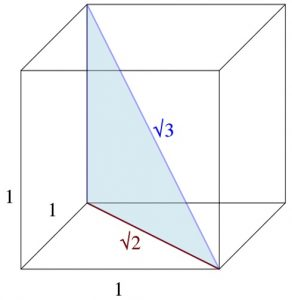Copy this diagram. Why do the diagonals of a unit cube have lengths √2 and √3?

To draw a cube first draw a square, then draw another square the same size that looks as if it is behind the first square, then join the vertices with four parallel lines.

What are the lengths of the diagonals of a cuboid with edges of length 1, 1 and 2?

What about a cuboid with edges of length 1, 2 and 3?

Use an open top cuboid shaped box. Calculate the lengths of its diagonals. Check your answers by cutting out two triangles to fit on the bottom of the box and a triangle, like the blue triangle in the picture, to stand vertically between them with its hypotenuse joining opposite vertices of the box.

What are the lengths of the diagonals of a cuboid with edges a, b and c?